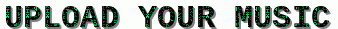# Free Bet Codes: SIMPLE MATHEMATICS ON 3 ODDS DAILY

#DAILY DOUBLING PROJECT✅💯💯💯

SIMPLE MATHEMATICS ON 3 ODDS DAILY

OUT OF 7 DAYS IN EVERY WEEK

MON👉10k X 3 odd s= 30k✅✅✅✅
TUE👉 10k X 3 odds = 30k ✅✅✅✅
WED👉10k X 3 odds = 30k ✅✅✅✅
THU👉 10k X 3 odds = 30k✅✅✅✅
FRI👉   10k X 3 odds = 30k♻️♻️♻️♻️
SAT👉  10k X 3 odds = 30k
SUN👉 10k X 3 odds = 30k

NOTE: this isn't a Rollover ✍

If you have 20k gain everyday for 7 days
Which gives you 140k.

Let's assume 2 days went wrong out of 7 days you will gain 100k✍

If you gaining 100k every week as your plan B
Isn't that enough for you?

@BET BIGGER WIN BIGGER